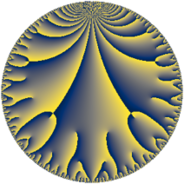# Properties

 Label 273.12.aLevel $273$ Weight $12$ Character orbit 273.a Rep. character $\chi_{273}(1,\cdot)$ Character field $\Q$ Dimension $132$ Newform subspaces $8$ Sturm bound $448$ Trace bound $2$

# Related objects

## Defining parameters

 Level: $$N$$ $$=$$ $$273 = 3 \cdot 7 \cdot 13$$ Weight: $$k$$ $$=$$ $$12$$ Character orbit: $$[\chi]$$ $$=$$ 273.a (trivial) Character field: $$\Q$$ Newform subspaces: $$8$$ Sturm bound: $$448$$ Trace bound: $$2$$ Distinguishing $$T_p$$: $$2$$

## Dimensions

The following table gives the dimensions of various subspaces of $$M_{12}(\Gamma_0(273))$$.

Total New Old
Modular forms 416 132 284
Cusp forms 408 132 276
Eisenstein series 8 0 8

The following table gives the dimensions of the cuspidal new subspaces with specified eigenvalues for the Atkin-Lehner operators and the Fricke involution.

$$3$$$$7$$$$13$$FrickeDim.
$$+$$$$+$$$$+$$$$+$$$$15$$
$$+$$$$+$$$$-$$$$-$$$$18$$
$$+$$$$-$$$$+$$$$-$$$$16$$
$$+$$$$-$$$$-$$$$+$$$$17$$
$$-$$$$+$$$$+$$$$-$$$$17$$
$$-$$$$+$$$$-$$$$+$$$$18$$
$$-$$$$-$$$$+$$$$+$$$$18$$
$$-$$$$-$$$$-$$$$-$$$$13$$
Plus space$$+$$$$68$$
Minus space$$-$$$$64$$

## Trace form

 $$132q + 92q^{2} + 149244q^{4} - 44712q^{6} - 67228q^{7} - 36156q^{8} + 7794468q^{9} + O(q^{10})$$ $$132q + 92q^{2} + 149244q^{4} - 44712q^{6} - 67228q^{7} - 36156q^{8} + 7794468q^{9} + 1216688q^{10} - 1081688q^{11} - 468504q^{12} - 4504276q^{14} - 6075000q^{15} + 150496276q^{16} - 2648456q^{17} + 5432508q^{18} - 6760008q^{19} - 16336404q^{21} + 66703056q^{22} - 55563116q^{23} - 248017464q^{24} + 1344850104q^{25} - 128876076q^{28} + 87758708q^{29} - 101848104q^{30} + 414181848q^{31} + 223571684q^{32} + 315373176q^{33} - 815770576q^{34} - 202557964q^{35} + 8812708956q^{36} - 742603824q^{37} - 2508626520q^{38} - 721793592q^{39} + 6922776136q^{40} + 1224527016q^{41} - 530005412q^{43} + 1757509528q^{44} + 1121858960q^{46} + 3519254832q^{47} - 959496192q^{48} + 37286732868q^{49} - 51352724q^{50} - 1011507912q^{51} - 6864464984q^{52} - 3949102268q^{53} - 2640198888q^{54} - 13202342848q^{55} - 19661164740q^{56} + 7138500192q^{57} - 29304997408q^{58} - 2641087176q^{59} - 3626775000q^{60} + 21025134064q^{61} - 38867698168q^{62} - 3969746172q^{63} + 149798650748q^{64} + 4935226556q^{65} - 12971400264q^{66} - 10630979584q^{67} + 43961659280q^{68} + 37649815416q^{69} - 11169528832q^{70} - 27467581344q^{71} - 2134975644q^{72} - 707925000q^{73} + 53360029160q^{74} - 1583609616q^{75} + 105813978256q^{76} + 53333048048q^{77} - 11548697472q^{78} - 30125108788q^{79} + 23041308104q^{80} + 460255540932q^{81} - 95973605616q^{82} - 157889330096q^{83} - 50185433088q^{84} - 325852146760q^{85} + 531116280496q^{86} + 48500492472q^{87} + 86644769712q^{88} + 164505999680q^{89} + 71844209712q^{90} - 49922571608q^{91} + 108534178672q^{92} - 27896920992q^{93} + 729004211496q^{94} + 166065914644q^{95} - 460576612944q^{96} + 149775610296q^{97} + 25987722908q^{98} - 63872594712q^{99} + O(q^{100})$$

## Decomposition of $$S_{12}^{\mathrm{new}}(\Gamma_0(273))$$ into newform subspaces

Label Dim. $$A$$ Field CM Traces A-L signs $q$-expansion
$$a_2$$ $$a_3$$ $$a_5$$ $$a_7$$ 3 7 13
273.12.a.a $$13$$ $$209.758$$ $$\mathbb{Q}[x]/(x^{13} - \cdots)$$ None $$-109$$ $$3159$$ $$-6095$$ $$218491$$ $$-$$ $$-$$ $$-$$ $$q+(-8-\beta _{1})q^{2}+3^{5}q^{3}+(567+20\beta _{1}+\cdots)q^{4}+\cdots$$
273.12.a.b $$15$$ $$209.758$$ $$\mathbb{Q}[x]/(x^{15} - \cdots)$$ None $$100$$ $$-3645$$ $$6095$$ $$-252105$$ $$+$$ $$+$$ $$+$$ $$q+(7-\beta _{1})q^{2}-3^{5}q^{3}+(1174-10\beta _{1}+\cdots)q^{4}+\cdots$$
273.12.a.c $$16$$ $$209.758$$ $$\mathbb{Q}[x]/(x^{16} - \cdots)$$ None $$-63$$ $$-3888$$ $$-3168$$ $$268912$$ $$+$$ $$-$$ $$+$$ $$q+(-4+\beta _{1})q^{2}-3^{5}q^{3}+(1298-4\beta _{1}+\cdots)q^{4}+\cdots$$
273.12.a.d $$17$$ $$209.758$$ $$\mathbb{Q}[x]/(x^{17} - \cdots)$$ None $$-10$$ $$4131$$ $$-6405$$ $$-285719$$ $$-$$ $$+$$ $$+$$ $$q+(-1+\beta _{1})q^{2}+3^{5}q^{3}+(1246-\beta _{1}+\cdots)q^{4}+\cdots$$
273.12.a.e $$17$$ $$209.758$$ $$\mathbb{Q}[x]/(x^{17} - \cdots)$$ None $$65$$ $$-4131$$ $$6405$$ $$285719$$ $$+$$ $$-$$ $$-$$ $$q+(4-\beta _{1})q^{2}-3^{5}q^{3}+(1071-4\beta _{1}+\cdots)q^{4}+\cdots$$
273.12.a.f $$18$$ $$209.758$$ $$\mathbb{Q}[x]/(x^{18} - \cdots)$$ None $$19$$ $$4374$$ $$-3168$$ $$302526$$ $$-$$ $$-$$ $$+$$ $$q+(1+\beta _{1})q^{2}+3^{5}q^{3}+(1354-\beta _{1}+\cdots)q^{4}+\cdots$$
273.12.a.g $$18$$ $$209.758$$ $$\mathbb{Q}[x]/(x^{18} - \cdots)$$ None $$36$$ $$-4374$$ $$3168$$ $$-302526$$ $$+$$ $$+$$ $$-$$ $$q+(2-\beta _{1})q^{2}-3^{5}q^{3}+(1060-3\beta _{1}+\cdots)q^{4}+\cdots$$
273.12.a.h $$18$$ $$209.758$$ $$\mathbb{Q}[x]/(x^{18} - \cdots)$$ None $$54$$ $$4374$$ $$3168$$ $$-302526$$ $$-$$ $$+$$ $$-$$ $$q+(3-\beta _{1})q^{2}+3^{5}q^{3}+(1147-2\beta _{1}+\cdots)q^{4}+\cdots$$

## Decomposition of $$S_{12}^{\mathrm{old}}(\Gamma_0(273))$$ into lower level spaces

$$S_{12}^{\mathrm{old}}(\Gamma_0(273)) \cong$$ $$S_{12}^{\mathrm{new}}(\Gamma_0(1))$$$$^{\oplus 8}$$$$\oplus$$$$S_{12}^{\mathrm{new}}(\Gamma_0(3))$$$$^{\oplus 4}$$$$\oplus$$$$S_{12}^{\mathrm{new}}(\Gamma_0(7))$$$$^{\oplus 4}$$$$\oplus$$$$S_{12}^{\mathrm{new}}(\Gamma_0(13))$$$$^{\oplus 4}$$$$\oplus$$$$S_{12}^{\mathrm{new}}(\Gamma_0(21))$$$$^{\oplus 2}$$$$\oplus$$$$S_{12}^{\mathrm{new}}(\Gamma_0(39))$$$$^{\oplus 2}$$$$\oplus$$$$S_{12}^{\mathrm{new}}(\Gamma_0(91))$$$$^{\oplus 2}$$